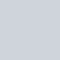# 先來看看今天的結果圖## -> 此篇文章的程式碼 github

Day03_圖片剪裁旋轉縮放_crop_rotate_resize.ipynb

# 1. 裁減圖片

``````def crop_img(img):

# left, right
x_l, x_r = 300, 700

# up, down
y_u, y_d = 0, 400

# crop image
crop_img = img[y_u:y_d, x_l:x_r]  # notice: first y, then x

return crop_img``````# 2. 旋轉圖片 cv2.getRotationMatrix2D

``````def rotate_img(img):
(h, w, d) = img.shape # 讀取圖片大小
center = (w // 2, h // 2) # 找到圖片中心

# 第一個參數旋轉中心，第二個參數旋轉角度(-順時針/+逆時針)，第三個參數縮放比例
M = cv2.getRotationMatrix2D(center, 15, 1.0)

# 第三個參數變化後的圖片大小
rotate_img = cv2.warpAffine(img, M, (w, h))

return rotate_img``````# 3. 縮放圖片 cv2.resize

``````def resize_img(img):

scale_percent = 50 # 要放大縮小幾%
width = int(img.shape * scale_percent / 100) # 縮放後圖片寬度
height = int(img.shape * scale_percent / 100) # 縮放後圖片高度
dim = (width, height) # 圖片形狀
resize_img = cv2.resize(img, dim, interpolation = cv2.INTER_AREA)

return resize_img```````jupyter notebook` 中，直接顯示的圖片結果看不出大小差異，

# Reference